# Practice test

 Date 28.05.2018 Size 124.01 Kb. #52217
8  Kinetics & Equilibrium

PRACTICE TEST

1. Which of the following does NOT influence the speed of a chemical reaction?

a) concentration of reactants

b) nature of reactants

c) temperature

d) none of these
2. What would cause the change in the kinetic energy diagrams as shown?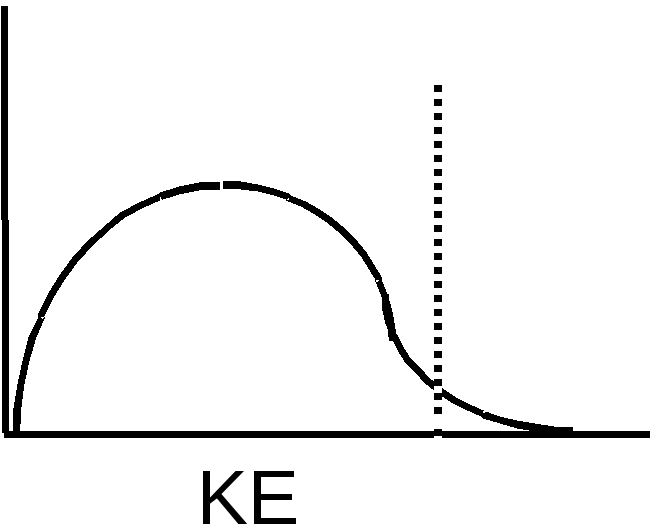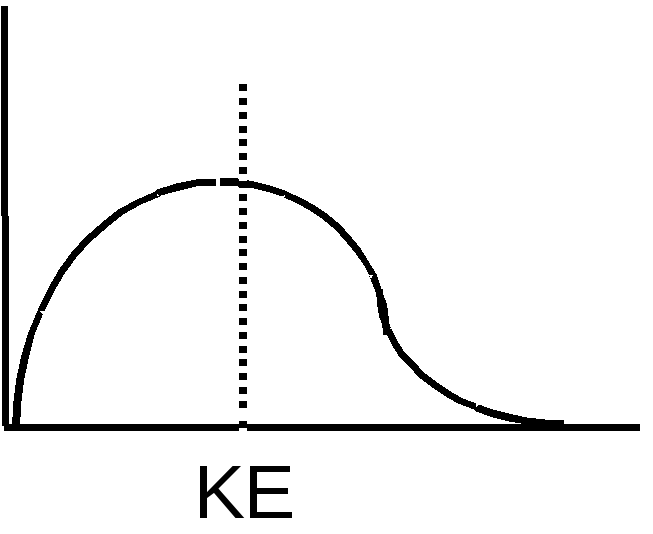a) increasing the H

b) decreasing the temperature

c) increasing the surface area

3. A time vs. concentration graph is presented below for the reaction A  B. What is the rate of appearance of ‘B’ 20 seconds after the start of the reaction?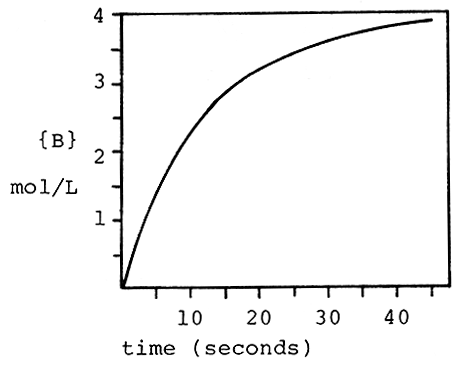a) 0.050 mol/Ls c) 0.010 mol/Ls

b) 3.2 mol/Ls d) 9.8 mol/Ls

4. The reaction 3O2  2O3 is proceeding with a rate of disappearance of O2 equal to 0.60 mol/Ls. What is the rate of appearance of O3, in mol/Ls?

a) 0.60 b) 0.40 c) 0.90 d) 0.10

5. A reaction has the rate law Rate = k[A]2[B]. What is the overall order of the reaction?

a) 0 b) 1 c) 2 d) 3

6. What are the correct units for a second order rate constant?

a) mol/Ls c) L/mols

b) 1/s d) mol2/L2s

7. The reaction I- + OCl-  IO- + Cl- is first order with respect to I- and first order with respect to OCl-. The rate constant is 6.1 x 10-2 L/mols. What is the rate of reaction when [I-] = 0.10 M and [OCl-] = 0.20 M?

a) 2.4 x 10-4 M/s c) 1.2 x 10-4 M/s

b) 1.2 x 10-3 M/s d) 2.4 x 10-5 M/s
8. A reaction and its rate law are given below. When [C4H6] = 2.0 M, the rate is 0.106 M/s.

What is the rate when [C4H6] = 4.0 M?

2 C4H6  C8H12 Rate = k[C4H6]2

a) 0.053 M/s c) 0.424 M/s

b) 0.212 M/s d) 0.022 M/s

9. The rate law for the reaction

2NO(g) + O2(g)  2NO2(g)

is Rate = k[NO]2[O2]. What happens to the rate when the concentration of NO is doubled?

a) the rate doubles c) the rate is halved

b) the rate quadruples d) none of these

10. Below is some rate data for the hypothetical reaction, 2A + B  C. What is the rate law for this reaction?

 Experiment [A]o [B]o Rate (M/s) 1 2.0 M 1.0 M 0.100 2 2.0 M 2.0 M 0.400 3 4.0 M 1.0 M 0.100

a) Rate = k[A][B] c) Rate = k[A]2[B]2

b) Rate = k[A]2[B] d) Rate = k[B]2

11. The acid catalyzed decomposition of hydrogen peroxide is a first order reaction with the rate constant given below. For an experiment in which the starting concentration of hydrogen peroxide is 0.110 M, what is the concentration of H2O2 450 minutes after the reaction begins?

2H2O2  2H2O + O2 k=1.33 x 10-4 min-1

a) 0.0961 M c) 0.00658 M

b) 0.104 M d) 0.0156 M
12. What is the rate constant for a first order reaction for which the half-life is 85.0 sec?

a) 0.00814 sec-1 d) 0.0118 sec-1

b) 4.44 sec-1 e) 58.9 sec-1

13. What fraction of a reactant remains after 3 half-lives of a first order reaction?

a) 1/2 c) 1/8

b) 1/3 d) 1/12
14. Assume a reaction occurs by the mechanism given below. What is the rate law for the reaction?

A + B  C fast

C  D slow

a) Rate = k[A][B][C]

b) Rate = k[A]2

c) Rate = k[A][B]

d) Rate = k[A][B]/[D]

15. According to collision theory, which of the following factors does NOT influence the rate of reaction?

a) collision frequency

b) collision energy

d) collision rebound direction
16. What distance corresponds to the activation energy for the reaction of X to Y?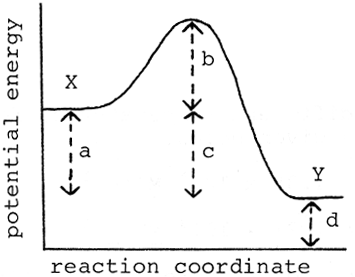a) a b) b c) c d) d

17. At what point on the potential energy diagram given below does the transition state (activated complex) occur?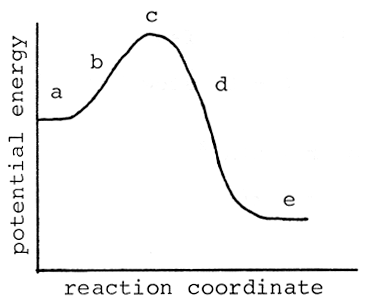a) a c) c

b) b d) e

18. Which of the following is NOT true about a catalyst?

a) it speeds up the forward reaction

b) is acts as an inhibitor

c) it speeds up the reverse reaction

d) it may be heterogeneous

19. Consider the reaction system,

CoO(s) + H2(g) Co(s) + H2O(g).

The equilibrium constant expression is

a)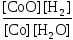c)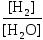b)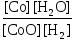d)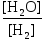20. Given the equilibrium,

2SO2(g) + O2(g) 2SO3(g), if this equilibrium is established by beginning with equal number of moles of SO2 and O2 in a 1.0 Liter bulb, then the following must be true at equilibrium:

a) [SO2] = [SO3] c) [SO2] < [O2]

b) 2[SO2] = 2[SO3] d) [SO2] > [O2]

Questions 21 & 22 refer to the following:

At a given temperature, 0.300 mole NO, 0.200 mol Cl2 and 0.500 mol ClNO were placed in a 25.0 Liter container. The following equilibrium is established: 2ClNO(g) 2NO(g) + Cl2(g)

21. At equilibrium, 0.600 mol of ClNO was present. The number of moles of Cl2 present at equilibrium is

a) 0.050 b) 0.100 c) 0.150 d) 0.200

22. The equilibrium constant, Kc, is:

a) 4.45 x 10-4 c) 0.167

b) 6.67 x 10-4 d) 1500
23. At 985C, the equilibrium constant for the reaction,

H2(g) + CO2(g) H2O(g) + CO(g)

is 1.63. What is the equilibrium constant for the reverse reaction?

a) 1.63 c) 0.613

b) 0.815 d) 1.00

24. What is the relationship between Kp and Kc for the reaction, 2ICl(g) I2(g) + Cl2(g)?

a) Kp = Kc(RT)-1 c) Kp = Kc

b) Kp = Kc(RT) d) Kp = Kc(2RT)

25. For the reaction 2NO2(g) N2O4(g), Kp at 25C is 7.3, when all partial pressures are expressed in atmospheres. What is Kc for this reaction? [R=0.0821 Latmmol-1K-1]

a) 4270 c) 179

b) 0.0119 d) 2.06
26. 0.200 mol NO is placed in a one liter flask at 2273 K. After equilibrium is attained, 0.0863 mol N2 and 0.0863 mol O2 are present. What is Kc for this reaction?

2NO(g) N2(g) + O2(g)

a) 9.92 d) 39.7

b) 3.15 e) 0.576

27. N2O4(g) 2 NO2(g)

At 25C, 0.11 mole of N2O4 reacts to form 0.10 mol of N2O4 and 0.02 mole of NO2. At 90C, 0.11 mole of N2O4 forms 0.050 mole of N2O4 and 0.12 mole of NO2. From these data we can conclude

a) N2O4 molecules react by a second order rate law.

b) N2O4 molecules react by a first order rate law.

c) the reaction is exothermic.

d) the equilibrium constant for the reaction above increases with an increase in temperature.

28. For the equilibrium system

H2O(g) + CO(g) H2(g) + CO2(g)

H = -42 kJ/mol

Kc equals 0.62 at 1260 K. If 0.10 mole each of H2O, CO, H2 and CO2 (each at 1260 K) were placed in a 1.0-Liter flask at 1260 K, when the system came to equilibrium…
 The temperature would The mass of CO would a) decrease increase b) decrease decrease c) remain constant increase d) increase decrease

N2(g) + 3H2(g) 2NH3(g) + heat

the conditions that would favor maximum conversion of the reactants to products would be

a) high temperature and high pressure

b) high temperature, pressure unimportant

c) high temperature and low pressure

d) low temperature and high pressure

30. Solid HgO, liquid Hg, and gaseous O2 are placed in a glass bulb and are allowed to reach equilibrium at a given temperature.

2HgO(s) 2Hg(l) + O2(g) H = +43.4 kcal

The mass of HgO in the bulb could be increased by

b) removing some O2.

c) reducing the volume of the bulb.

d) increasing the temperature.